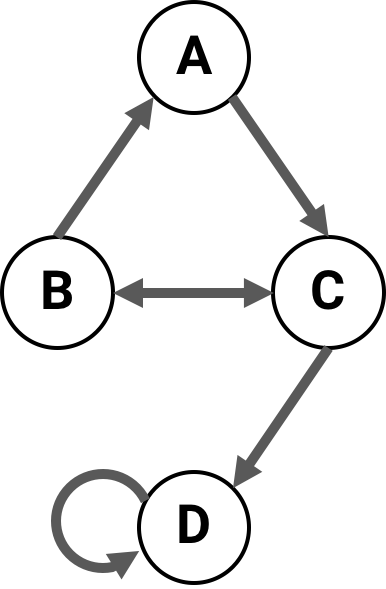# Connectivity matrix conventions¶

Throughout PyPhi, if $$CM$$ is a connectivity matrix, then $$[CM]_{i,j} = 1$$ means that there is a directed edge $$(i,j)$$ from node $$i$$ to node $$j$$, and $$[CM]_{i,j} = 0$$ means there is no edge from $$i$$ to $$j$$.

For example, this network of four nodeshas the following connectivity matrix:

>>> cm = [[0, 0, 1, 0],
...       [1, 0, 1, 0],
...       [0, 1, 0, 1],
...       [0, 0, 0, 1]]


# Transition probability matrix conventions¶

There are several ways to write down a transition probability matrix (TPM). With both state-by-state and state-by-node TPMs, one is confronted with a choice about which rows correspond to which states. In state-by-state TPMs, this choice must also be made for the columns.

Either the first node changes state every other row (LOLI):

State at $$t$$ $$P(N = 1)$$ at $$t+1$$
A, B A B
(0, 0) 0.1 0.2
(1, 0) 0.3 0.4
(0, 1) 0.5 0.6
(1, 1) 0.7 0.8

Or the last node does (HOLI):

State at $$t$$ $$P(N = 1)$$ at $$t+1$$
A, B A B
(0, 0) 0.1 0.2
(0, 1) 0.5 0.6
(1, 0) 0.3 0.4
(1, 1) 0.7 0.8

Note that the index $$i$$ of a row in a TPM encodes a network state: convert the index to binary, and each bit gives the state of a node. The question is, which node?

Throughout PyPhi, we always choose the first convention—the state of the first node (the one with the lowest index) varies the fastest. So, the lowest-order bit—the one’s place—gives the state of the lowest-index node.

We call this convention the LOLI convention: Low Order bits correspond to Low Index nodes. The other convention, where the highest-index node varies the fastest, is similarly called HOLI.

The rationale for this choice of convention is that the LOLI mapping is stable under changes in the number of nodes, in the sense that the same bit always corresponds to the same node index. The HOLI mapping does not have this property.

Note

This applies to only situations where decimal indices are encoding states. Whenever a network state is represented as a list or tuple, we use the only sensible convention: the $$i^{\textrm{th}}$$ element gives the state of the $$i^{\textrm{th}}$$ node.

Tip

There are various conversion functions available for converting between TPMs, states, and indices using different conventions: see the pyphi.convert module.